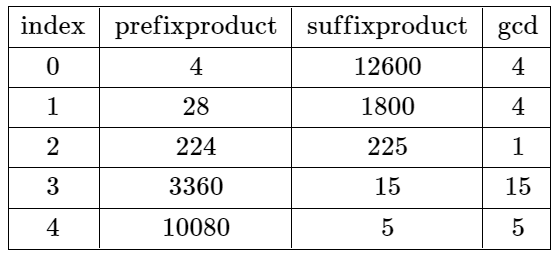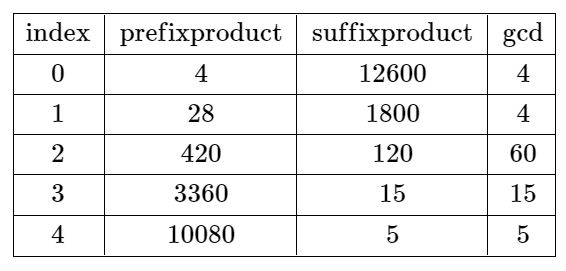2584. Split the Array to Make Coprime Products
Hard
240
104

You are given a 0-indexed integer array `nums` of length `n`.

A split at an index `i` where `0 <= i <= n - 2` is called valid if the product of the first `i + 1` elements and the product of the remaining elements are coprime.

• For example, if `nums = [2, 3, 3]`, then a split at the index `i = 0` is valid because `2` and `9` are coprime, while a split at the index `i = 1` is not valid because `6` and `3` are not coprime. A split at the index `i = 2` is not valid because `i == n - 1`.

Return the smallest index `i` at which the array can be split validly or `-1` if there is no such split.

Two values `val1` and `val2` are coprime if `gcd(val1, val2) == 1` where `gcd(val1, val2)` is the greatest common divisor of `val1` and `val2`.

Example 1:```Input: nums = [4,7,8,15,3,5]
Output: 2
Explanation: The table above shows the values of the product of the first i + 1 elements, the remaining elements, and their gcd at each index i.
The only valid split is at index 2.
```

Example 2:```Input: nums = [4,7,15,8,3,5]
Output: -1
Explanation: The table above shows the values of the product of the first i + 1 elements, the remaining elements, and their gcd at each index i.
There is no valid split.
```

Constraints:

• `n == nums.length`
• `1 <= n <= 104`
• `1 <= nums[i] <= 106`
Accepted
8.4K
Submissions
38.3K
Acceptance Rate
22.0%

Seen this question in a real interview before?
1/4
Yes
No

Discussion (0)

Related Topics